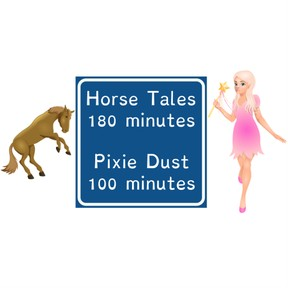Converting hours and minutes

# Converting hours and minutes

I can convert time between hours and minutes.

No account needed.8,000 schools use Gynzy92,000 teachers use Gynzy1,600,000 students use Gynzy

## General

Students will learn strategies to convert hours to minutes and minutes to hours. They are encouraged to think about when this would be useful in everyday life.

## Standards

CCSS.Math.Content.3.MD.A.1

## Learning objective

Students will be able to convert time between hours and minutes.

## Introduction

In a discussion about movie runtimes, students are asked to consider when it would be helpful to know how many hours long the movie would be. For example, if you had to catch a bus and only had two hours, etc.

## Instruction

The lesson starts with covering basic prerequisite clock skills such as counting the minutes by 5 around the clock and counting the quarter hours. Students are encouraged to clap out the pattern of 15, 30, 45, 60 for the quarter hours and also move the hands on a clock as they say the pattern. Students then practice adding quarter hours. Next, they practice multiplying and dividing by 60 in advance to learning the strategy to convert minutes to hours. Step-by-step instruction and practice follow. The lesson concludes with several problem-solving activities. Circling back to the beginning, the movie runtimes are converted to solve the problem of picking a movie that is less than two hours.

## Quiz

Students respond to ten multiple-choice and true/false questions.

## Closing

Students will compete in a game matching time in minutes to times in hours and minutes.

### The online teaching platform for interactive whiteboards and displays in schools

• Save time building lessons

• Manage the classroom more efficiently

• Increase student engagement